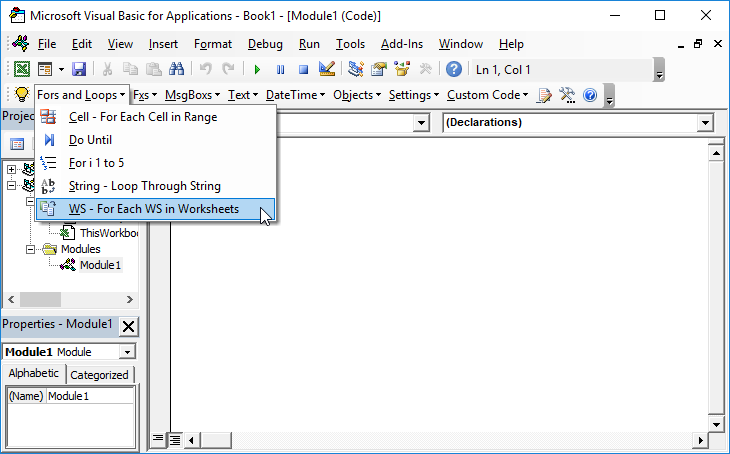# VBA – Sort Sheets Alphabetically

The following routine will sort the sheets in a workbook alphabetically. The flag “Sort_Mode_Descending” can be switched between descending and ascending as required. The routine is case INSENSITIVE.

``````Sub Sort_Sheets()
Dim Sort_Mode_Descending As Boolean
Dim No_of_Sheets As Integer
Dim Outer_Loop As Integer
Dim Inner_Loop As Integer
No_of_Sheets = Sheets.Count
'Change Flag As appropriate
Sort_Mode_Descending = False
For Outer_Loop = 1 To No_of_Sheets
For Inner_Loop = 1 To Outer_Loop
If Sort_Mode_Descending = True Then
If UCase(Sheets(Outer_Loop).Name) > UCase(Sheets(Inner_Loop).Name) Then
Sheets(Outer_Loop).Move Before:=Sheets(Inner_Loop)
End If
End If
If Sort_Mode_Descending = False Then
If UCase(Sheets(Outer_Loop).Name) < UCase(Sheets(Inner_Loop).Name) Then
Sheets(Outer_Loop).Move Before:=Sheets(Inner_Loop)
End If
End If

Next Inner_Loop
Next Outer_Loop``````